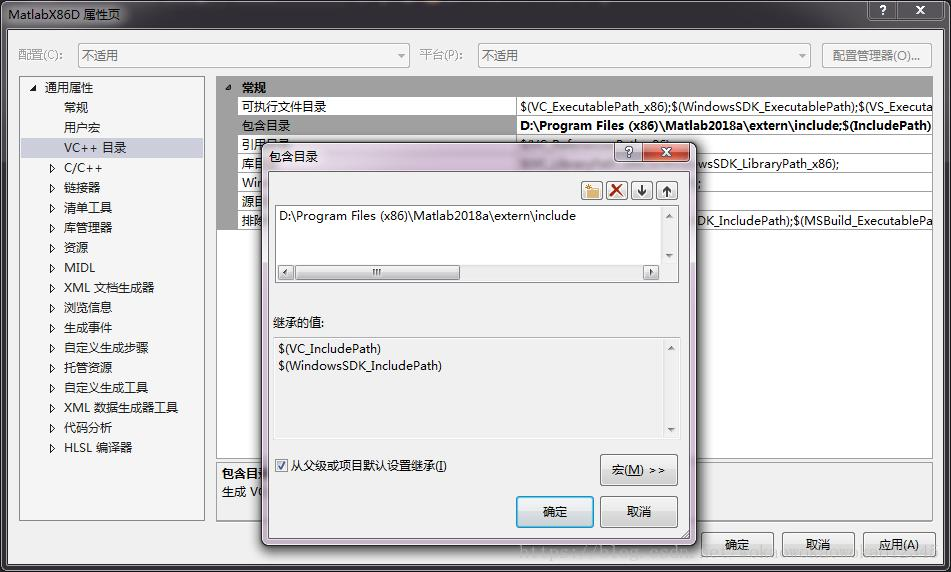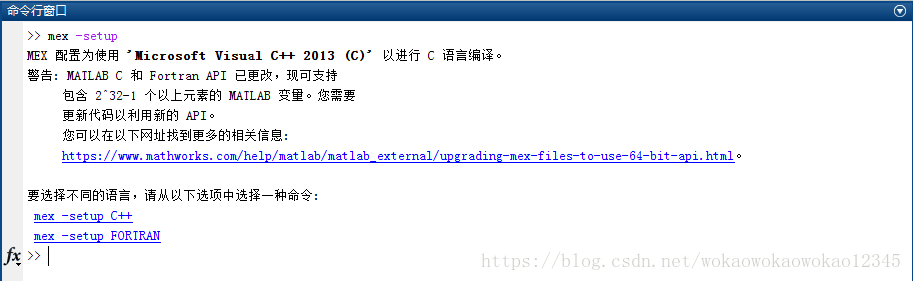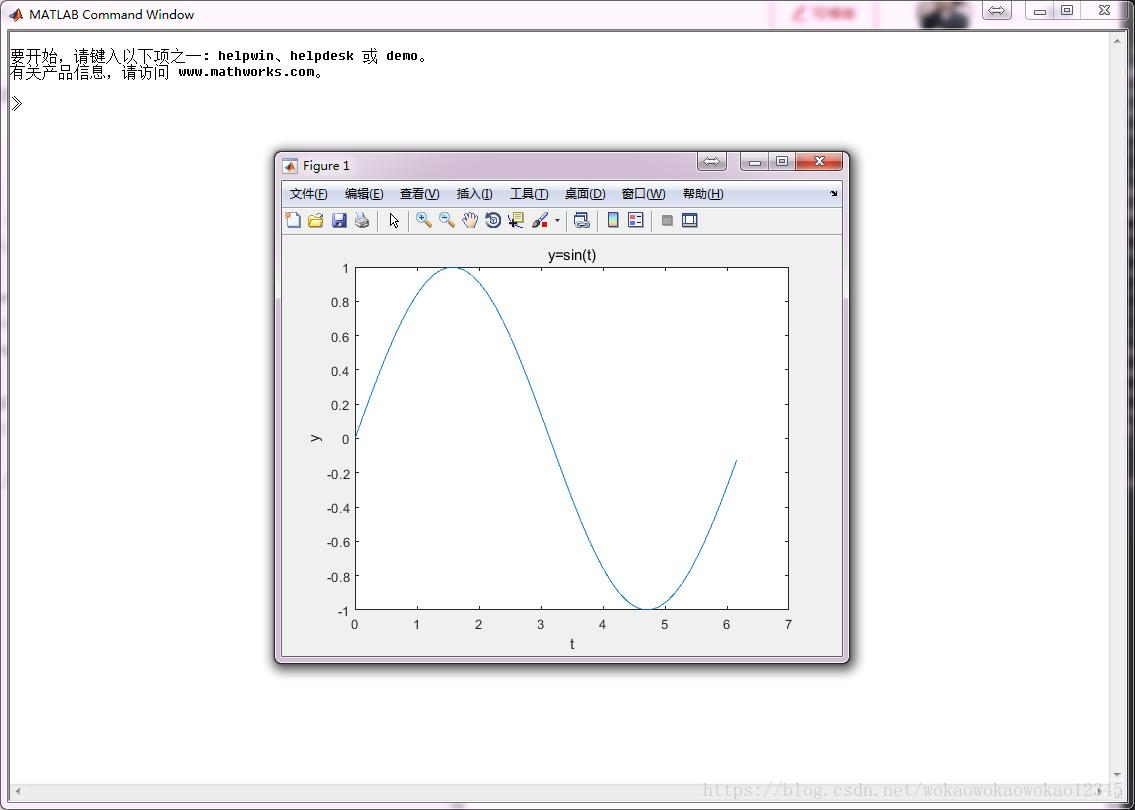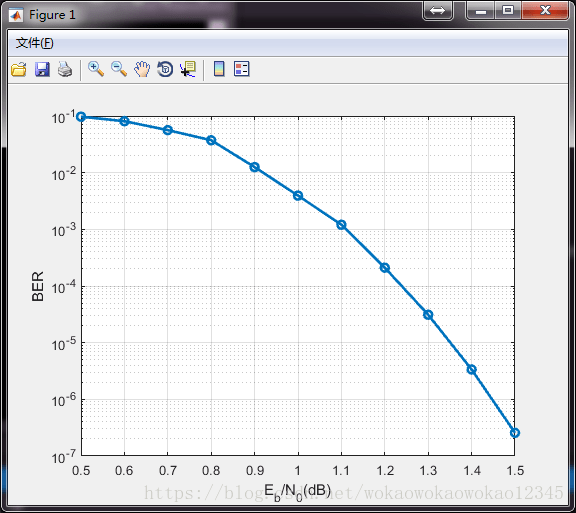# C++ MATLAB mixed programming – VS project calls MATLAB function DLL

## Introduction

MATLAB is undoubtedly a powerful matrix operation and data analysis software, which integrates many functions inside. It will bring great convenience to our data analysis research if we can call related functions when programming in C++. Here is a summary of how to call MATLAB functions in VS projects, and how to solve the problems encountered.

## VS Configuration

This step is very important, similar to other C++ library configurations, such as: add include directories and library paths.
Add library directory (find in your own MATLAB installation path): D:\Program Files (x86)\Matlab2018a\extern\includeAdd library path (find in your own MATLAB installation path): D:\Program Files (x86)\Matlab2018a\extern\lib\win64\microsoft## Configure system environment variables

Add the following three items to “Path” in the system variable in the Windows environment variable:
D:\Program Files (x86)\Matlab2018a\bin; (it already exists after installing MATLAB, no need to add it)
D:\Program Files (x86)\Matlab2018a\bin\win32;
D:\Program Files (x86)\Matlab2018a\bin\win64## MATLAB Configuration

Enter in the command window of Matlab: mex -setup
select: mex -setup C++Then enter: mbuild -setup
Select: mex -setup C++ -client MBUILDend of configuration## Test

We will call MATLAB in the C ++ project of VS2013. There are two calling methods: (1) calling the MATLAB engine; (2) calling the DLL generated by the MATLAB function.

### Method 1 calls MATLAB engine

Then, the reference function calls the dependent library (lib) libeng.lib libmx.lib libmat.lib, and then the MATLAB engine can be called.

```// import necessary lib
#pragma comment( lib, "libeng. lib")
#pragma comment( lib, "libmx. lib")
#pragma comment( lib, "libmat. lib")```

MATLAB engine calls are similar to file operations: open and close operations.
Turn on the engine:

```Engine *ep;
if (!(ep = engOpen("\0")))
{
fprintf(stderr, "\\
Can't start MATLAB engine\\
");
return EXIT_FAILURE;
} ```

Define variables need to be converted to matlab format. For example, use: mxCreateDoubleMatrix and memcpy functions.
To pass data into the engine use: engPutVariable(). Then call engEvalString(ep, “matlab instruction”) to operate with matlab instructions as parameters. Cleanup is required after calculation operations are completed: the mxDestroyArray() function cleans up the variables generated by mxCreateDoubleMatrix.
engClose() closes the engine.

Complete drawing example:

```#include <stdlib.h>
#include <stdio.h>
#include <string.h>

// import necessary lib
#pragma comment( lib, "libeng. lib")
#pragma comment( lib, "libmx. lib")
#pragma comment( lib, "libmat. lib")

int main(void)
{
Engine *ep;

// open engine
if (!(ep = engOpen("\0")))
{
fprintf(stderr, "\\
Can't start MATLAB engine\\
");
return EXIT_FAILURE;
}

// generate variables
int Nsample = 50;
const double PI = 3.1415926;
double *t = new double[Nsample];

for (int i = 0; i < Nsample; i ++ )
{
t[i] = i * 2 * PI / Nsample;
}

mxArray *T = NULL, *result = NULL;
T = mxCreateDoubleMatrix(1, Nsample, mxREAL);
memcpy((void *)mxGetPr(T), (void *)t, Nsample*sizeof(t));

engPutVariable(ep, "T", T); // put data to engine

// execute matlab operations
engEvalString(ep, "Y=sin(T);");
engEvalString(ep, "plot(T,Y);");
engEvalString(ep, "title('y=sin(t)');");
engEvalString(ep, "xlabel('t');");
engEvalString(ep, "ylabel('y');");

");
fgetc(stdin);

// clean operation(don't forget!!!)
mxDestroyArray(T);
engEvalString(ep, "close;");

// close engine
engClose(ep);

return EXIT_SUCCESS;
}```

Effect:Error solution:

```1>------ Build started: Project: CMatlab, Configuration: Debug Win32 ------
1>main.obj : error LNK2019: unresolved external symbol _mxGetPr_800 referenced in function _main
1>main.obj : error LNK2019: unresolved external symbol _mxDestroyArray_800, which is referenced in function _main
1>main.obj : error LNK2019: unresolved external symbol _mxCreateDoubleMatrix_800 referenced in function _main
1>main.obj : error LNK2019: unresolved external symbol _engEvalString referenced in function _main
1>main.obj : error LNK2019: unresolved external symbol _engOpen referenced in function _main
1>main.obj : error LNK2019: unresolved external symbol _engClose referenced in function _main
1>main.obj : error LNK2019: unresolved external symbol _engPutVariable referenced in function _main
1>E:\shizhenwei\VS2013Projects\CMatlab\Debug\CMatlab.exe : fatal error LNK1120: 7 unresolved external commands```

The problem is compatibility. We need to change the vs project to 64-bit, and then it can be solved.

### Method 2 calls the DLL generated by MATLAB

Calling the DLL generated by MATLAB is a more general method, so that you only need to call this module every time you use it.
First, the function that needs to be completed by MATLAB is made into a MATLAB function.
Then, use the mcc command to compile to get h, dll, lib and other files, and you only need to add the above files in the C/C++ project.

MATLAB function code

```function showBER(SNR_indB,BER)
semilogy(SNR_indB,BER,'-o','linewidth',2);
grid on
xlabel('E_b/N_0(dB)');
ylabel('BER');```

After compiling, enter on the MATLAB command line:

`mcc -B csharedlib:showBER showBER.m`

get a set of filesCopy the .dll .h .lib files to the C project, and add the following code to the cpp file that needs to call the drawing module:

```#include "showBER.h"

#pragma comment( lib, "libeng. lib" )
#pragma comment( lib, "libmx. lib" )
#pragma comment( lib, "libmat. lib" )
#pragma comment( lib, "mclmcrrt.lib" )
#pragma comment( lib, "showBER.lib" )

void main(void)
{
double SNR[] = { 0.5, 0.6, 0.7, 0.8, 0.9, 1.0, 1.1, 1.2, 1.3, 1.4, 1.5 };
double BER[] = { 9.728816e-002, 8.099609e-002, 5.633803e-002, 3.733608e-002, 1.253970e-002, 3.936489e-003, 1.206820e-003, 2.1040 52e-004, 3.109879e-005 , 3.365857e-006, 2.565067e-007 };
int len = sizeof(SNR) / sizeof(SNR);

showBERInitialize();

mxArray* xSNR = mxCreateDoubleMatrix(1, len, mxREAL);
memcpy(mxGetPr(xSNR), (void*)SNR, sizeof(SNR));
mxArray* xBER = mxCreateDoubleMatrix(1, len, mxREAL);
memcpy(mxGetPr(xBER), (void*)BER, sizeof(BER));

mlfShowBER(xSNR, xBER);
system("PAUSE");

showBERTerminate();
}```

running result### Hint

For other packaging methods, please refer to the introductions in links 3 and 4, and personal testing is available.

refer to
The method of calling matlab function in C/C++ VS_vs calling matlab function_AI eat big melon blog-CSDN blog
http://blog.163.com/rongting_chen/blog/static/164906844201252354518462/
https://www.cnblogs.com/jmliao/p/5575202.html
Example of C++ and matlab R2014 mixed programming method under 64-bit system vs2010 platform_add exported functions_Jerry-1990’s Blog-CSDN Blog